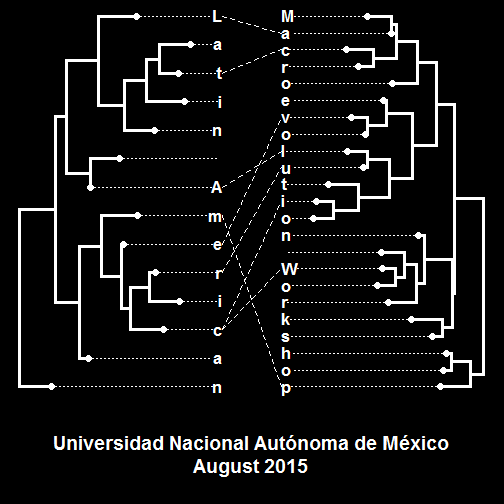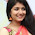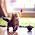## Wednesday, August 19, 2015

### Logo for UNAM course in R

I will be teaching a 4-day workshop at Universidad Nacional Autónoma de Mexico (UNAM) in Mexico City with Alejandro Gonzalez-Voyer next week. Here is our logo design for the course (generated in R):

``````library(phytools)
``````
``````## Loading required package: ape
``````
``````set.seed(499)
tips1<-strsplit("Latin American","")[]
tips2<-strsplit("Macroevolution Workshop","")[]
tr1<-rtree(n=length(tips1))
tr1\$tip.label<-tips1[length(tips1):1]
tr2<-rtree(n=length(tips2))
tr2\$tip.label<-tips2[length(tips2):1]
par(bg="black")
par(fg="white")
assoc<-cbind(
c("L","t","e","A","r","c","c","m"),
c("a","c","v","l","u","W","i","p"))
plot.new()
par(font=2)
obj<-cophylo(tr1,tr2,assoc=assoc,rotate=FALSE)
layout(mat=matrix(c(1,2),2,1),heights=c(0.8,0.2))
plot(obj,fsize=1.4,lwd=3,ftype="b")
plot.new()
col="white",cex=1.6,font=2)
``````That's it!

1.2.